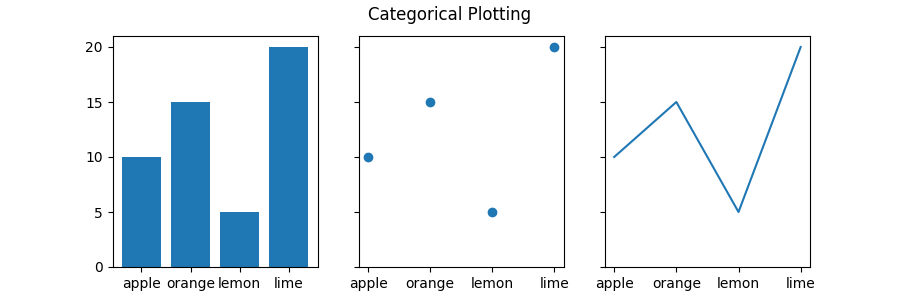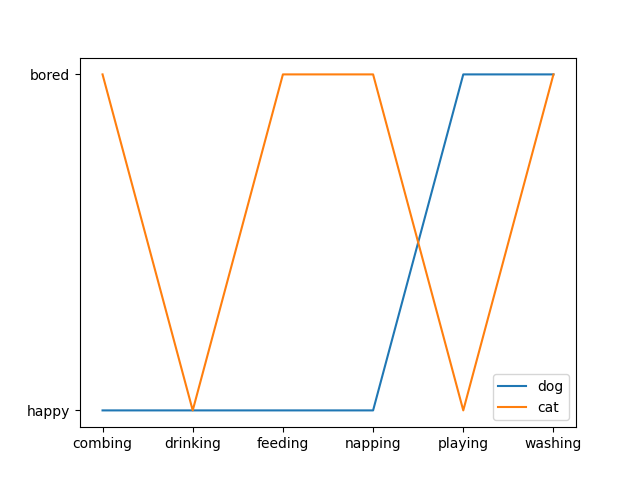# Plotting categorical variables¶

You can pass categorical values (i.e. strings) directly as x- or y-values to many plotting functions:

import matplotlib.pyplot as plt

data = {'apple': 10, 'orange': 15, 'lemon': 5, 'lime': 20}
names = list(data.keys())
values = list(data.values())

fig, axs = plt.subplots(1, 3, figsize=(9, 3), sharey=True)
axs.bar(names, values)
axs.scatter(names, values)
axs.plot(names, values)
fig.suptitle('Categorical Plotting')Out:

Text(0.5, 0.98, 'Categorical Plotting')


This works on both axes:

cat = ["bored", "happy", "bored", "bored", "happy", "bored"]
dog = ["happy", "happy", "happy", "happy", "bored", "bored"]
activity = ["combing", "drinking", "feeding", "napping", "playing", "washing"]

fig, ax = plt.subplots()
ax.plot(activity, dog, label="dog")
ax.plot(activity, cat, label="cat")
ax.legend()

plt.show()Total running time of the script: ( 0 minutes 1.031 seconds)

Keywords: matplotlib code example, codex, python plot, pyplot Gallery generated by Sphinx-Gallery